# Learn and Practise Writing the Numbers to 10

Learn and Practise Writing the Numbers to 10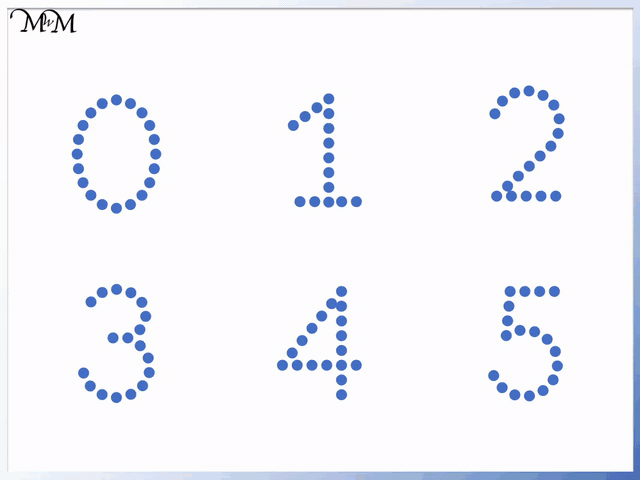• Here are the numbers from 0 to 10.
• It is helpful to learn the numbers to 5 and then learn the numbers from 6 to 10.
• Start to learn the numbers by tracing over them.
• As you become more familiar with the shape of each number, start to remove the tracing pattern and try drawing the numbers independently.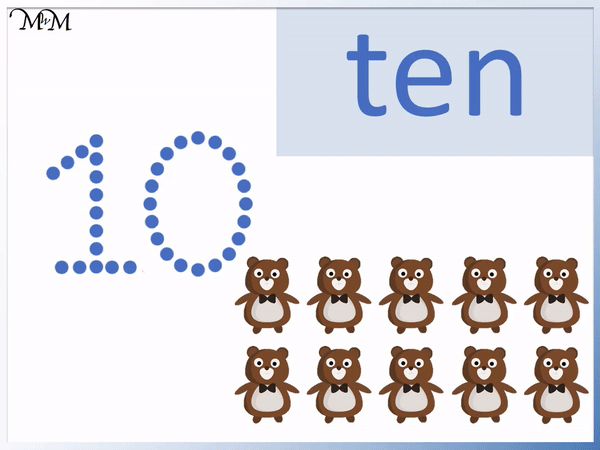• The number ten is made up of two digits: one and zero.
• The arrows show the direction and order to draw each number.
• Trace each digit by drawing over the dots in the correct order.
• 1 is drawn with a diagonal line upwards to the right and then a vertical line downwards, without taking the pencil off the page.
• The base is drawn by taking the pen off the page and drawing a horizontal line from left to right.
• The 0 is drawn by making a counterclockwise loop from the top, all the way around back to the start.
• The zero is slightly taller than it is wide.#### Writing Numbers Accompanying Activity Sheets# Number Writing Practice to 10

## Teaching Writing Numbers

When starting to learn how to write each number, start by forming the correct shape of each number by drawing over the top of tracing worksheets. Eventually begin to remove the tracings and try to form the number without using anything to trace over.

It is important to focus on keeping the stroke order and direction of the number consistent. If the number is drawn in the wrong direction, it is important to correct it quickly, to prevent the wrong muscle memory from developing. Return to using the tracing worksheets if this occurs.

Trace each number using dotted tracing sheets provided, making sure that the lines of each number are drawn in the correct order. Watch the animations provided to see the order to write each number.

It is important to have a correct pencil grip. If your child can draw letters of the alphabet, then they should also be able to draw numbers. Tracing activities are also provided below to help practise the correct stroke order for each number without needing to hold a pencil.

## Writing the Number 0

To write the number 0, start with your pencil at the top, in the middle. Bring it down to the left and draw a circle back to the top in an anticlockwise direction. The circle should be taller than it is wide.

Here is the animation showing how to trace the number zero.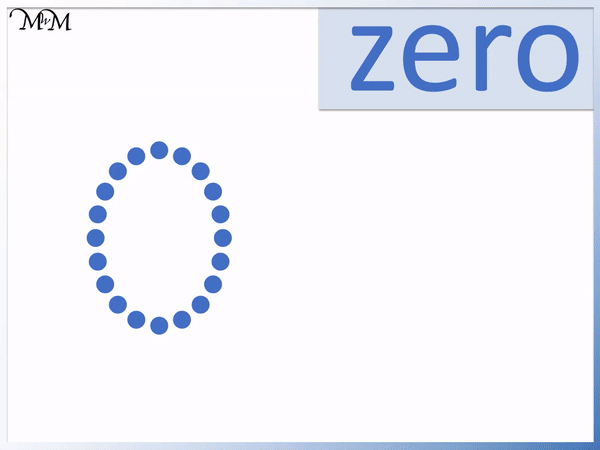Use the following tracing activity on your computer to practise the number zero.

## Writing the Number 1

The number 1 is drawn with 3 different straight lines. Start by drawing the first small line up to the right and immediately draw the second longer line coming vertically downwards without taking your pencil off the page. Then take your pencil off the page and draw the third line from left to right along the base of the number.

Here is the animation showing how to trace the number one.Use the following tracing activity on your computer to practise the number one.

## Writing the Number 2

The number 2 is drawn without taking the pencil off the page. Start by drawing a clockwise curve and then draw diagonally downwards to the left to draw a hook shape. Finally, move the pencil horizontally to the right to draw the base.

Here is the animation showing how to trace the number two.Use the following tracing activity on your computer to practise the number two.

## Writing the Number 3

To write the number 3, draw a clockwise loop but do not return fully to the beginning. Without taking the pencil off the page, come back horizontally to the right but this time, the clockwise loop will be drawn below the first clockwise loop. Again, do not complete this second loop.

Here is the animation showing how to trace the number three.Use the following tracing activity on your computer to practise the number three.

## Writing the Number 4

To write the number 4, start by drawing a line diagonally down to the left and then without taking the pencil off the page, draw a horizontal line from left to right. Take the pencil off the page and put it back where you started drawing. Draw a line vertically downwards, crossing through the horizontal line.

Here is the animation showing how to trace the number 4.Use the following tracing activity on your computer to practise the number four.

## Writing the Number 5

To write the number 5, start at the top left and draw a small line vertically downwards. Draw a loop clockwise until the pencil almost completes a full loop. Take the pencil off the page and place it at the top, at the starting point. Draw a horizontal line from left to right to draw the top of the 5.

Here is the animation showing how to trace the number 5.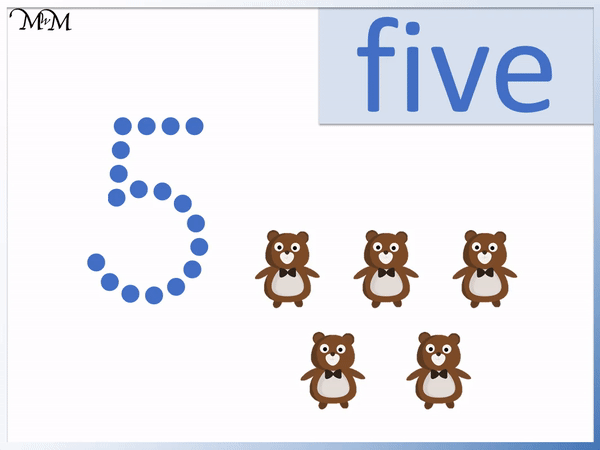Use the following tracing activity on your computer to practise the number five.

## Writing the Number 6

To write the number 6, start at the top right and draw an anticlockwise loop by moving the pencil down to the left. Continue the loop all the way around but it should finish mid-way down the first part of the loop. Do not take your pencil off the page when drawing the number 6.

Here is the animation showing how to trace the number 6.Use the following tracing activity on your computer to practise the number six.

## Writing the Number 7

To write the number 7, start in the top left and draw a horizontal line from left to right. This is the top of the seven. Without taking your pencil off the page, draw a line diagonally down to the left, finishing below the point that you started at.

Here is the animation showing how to trace the number 7.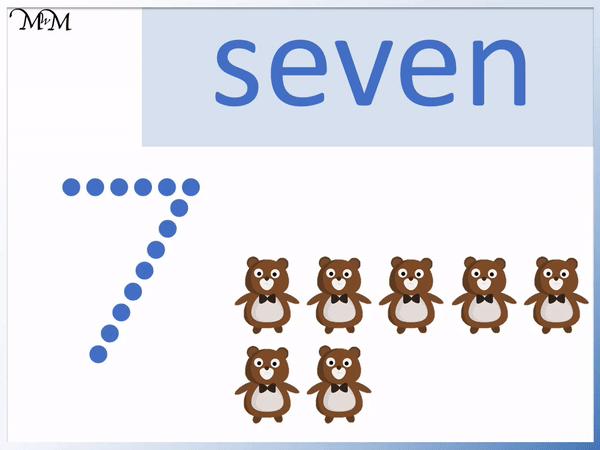Use the following tracing activity on your computer to practise the number seven.

## Writing the Number 8

To draw the number 8, start at the top middle and draw an ‘s’ shape. This is drawn by drawing a ‘c’ shape and then a backwards ‘c’ shape below it. Then complete the lower loop of the ‘s’ shape and finally, complete the upper loop of the ‘s’ shape. The number 8 is drawn without taking the pencil off the page.

We can draw an ‘s’ shape and then the hand motion is an ‘s’ shape in reverse. Once you have drawn an ‘s’ shape, you complete the bottom loop to get back to the middle and then complete the top loop to make the 8.

Here is the animation showing how to trace the number 8.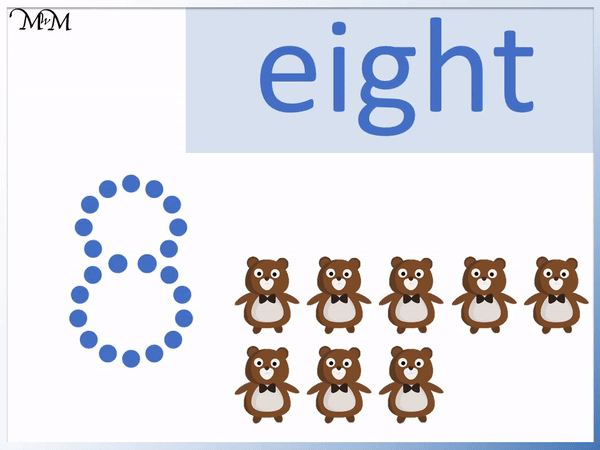Use the following tracing activity on your computer to practise the number eight.

## Writing the Number 9

To write the number 9, start at the top right and draw a counterclockwise loop, completing a full loop. Then without taking the pencil off the page, draw a vertical line straight downwards.

Here is the animation showing how to trace the number 9.Use the following tracing activity on your computer to practise the number nine.

## Writing the Number 10

The number 10 is simply made up of the digits 1 and 0.

Every number is written using the digits from 0 to 9 above. Drawing larger numbers beyond 10 is a good way to practise these learning these digits.

Here is the animation showing how to trace the number 10.Use the following tracing activity on your computer to practise the number ten.Now try our lesson on Counting to Ten using a Number Line where we learn how to count objects up to ten.error: Content is protected !!